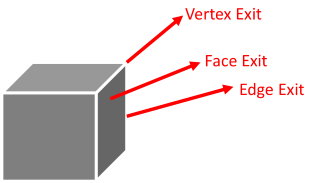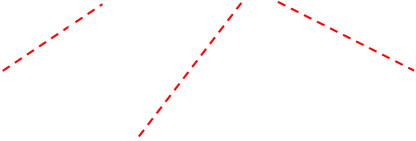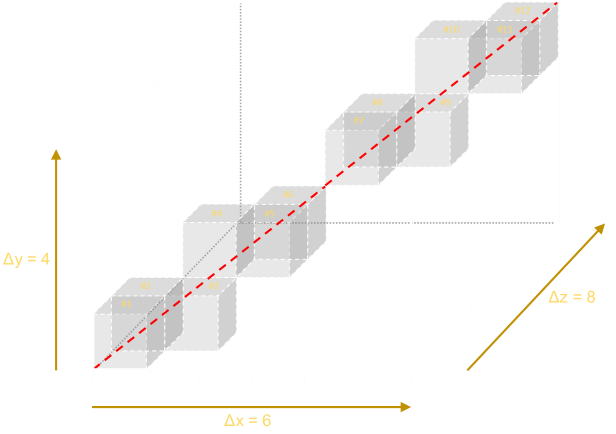﻿ Grid Puzzle# Grid Puzzle

 The other day I heard a little puzzle. At first it looked like it would be fairly trivial to solve, but I have to confess that it took me longer than I’d care to admit to come up with the solution. After coming up with the solution, I wrote a little interactive application (below), and feel that, if I’d had this before I started, I’d have come up with the solution quicker. Sometimes visualizations, simulations and models can really help in understanding a problem.### The PuzzleImagine there is a grid of squares. You draw a line connecting one intersection to another intersection on the grid. The question is: How many squares does this line cross through?
 What you need to derive to solve this puzzle is a function in which you input the Δx and Δy of the start and end of the line and it returns the number of cells that get split by the line. One way to imagine this is to think of the squares as cubes of jello. If you pointed a laser from one location to the other, how many of the cubes of jello does the laser pass through?### Try it out

Below is an interactive application you can experiment with to determine the relationship. Can you work it out?

Instructions: Click on the grid to draw a line connecting that location with the last location clicked, or alternatively, drag to make a line between two locations of your choice. The rubric in the top right gives the delta between the two coordinates, and the count of the number of squares bisected. What is the relationship between Δx, Δy and the count?

## Solution

The key to solving this puzzle is to realize that every time the laser goes into a square, it comes out again.

So, the number of boundaries it has to cross (and thus the number of cells that get visited) is the number of edges between the two coordinates. As a base line, the is Δx + Δy.

The laser will either go through a horizontal edge or the vertical edge of the grid on its way to the next cell, and each time this happens it increments, by one, the number of cells visited. However, there is a special case in which, if the laser goes exactly through a corner, it gets into a new cell by one fewer edges (visiting one less cell).Count = Δx + Δy - (Number of Corners passed through)

What we need to do is find out the number of times the line passes exactly through a corner.

When does the line pass through a corner? This happens when the coordinate pair delta have common factors (integer multiples). When the coordinate deltas are relatively prime, the line never passes through a corner, but for each common factor it does (for more background on this, check out prime factors).

The number of corners passed through is the Greatest Common Divisor of the two coordinate deltas.

### Solution

Count = Δx + Δy - GCD(Δx , Δy)

Pretty neat!Here's an examples with Δx=18 and Δy=4 Count = 18 + 4 - GCD(18,4) = 18 + 4 - 2 = 20 Answer: 20 squares are cut.

## 3D

With a little thinking, we can expand this formula to work in 3D. It's a little bit harder visualize, but imagine firing a laser from one corner of a rectangular slap of cubes to another corner. How many cubes will be passed through?Rather than two cases (edge or corner), there are three exit cases when we are dealing in 3d. The laser can exit the face, a cube edge, or a cube vertex.
 Let's imagine a laser being shot through opposite corners of a cuboid with dimensions: (2 x 3 x 4) How many cubes are sliced?If the laser exits a face, then it moves into a new cube, and we increment the number of cubes visited by one. If the laser exits the edge of a cube, then it's one fewer cubes that are cut.

We can determine the edges by looking at the 2D projections on the three axes (above), and we can reduce number of cubes correspondingly, and as a first blush of the final solution you might have been tempted to say this:

Count = Δx + Δy + Δz - GCD(Δx , Δy) - GCD(Δx , Δz) - GCD(Δy , Δz)

By using the GCD for each pair of axes, we can determine the number of edges passed through.

Can you see a problem with this? That's right we're over-discounting when the laser passes through a vertex. A vertex, by definition, is on multiple edges, so we need to add back in when the laser passes through the '3D corner' point.

Count = Δx + Δy + Δz - GCD(Δx , Δy) - GCD(Δx , Δz) - GCD(Δy , Δz) + GCD(Δx , Δy , Δz)

### Example

Here's a cuboid with Δx = 6, Δy = 4, Δz = 8Count = 6 + 4 + 8 - GCD(6,4) - GCD(6,8) - GCD(4,8) + GCD(4,6,8) Count = 6 + 4 + 8 - 2 - 2 - 4 + 2 Count = 12 This is the answer we expected! Jello is also pretty tasty to eat too (with or without a laser garnish).Image: stevendepolo

You can find a complete list of all the articles here.Click here to receive email alerts on new articles.